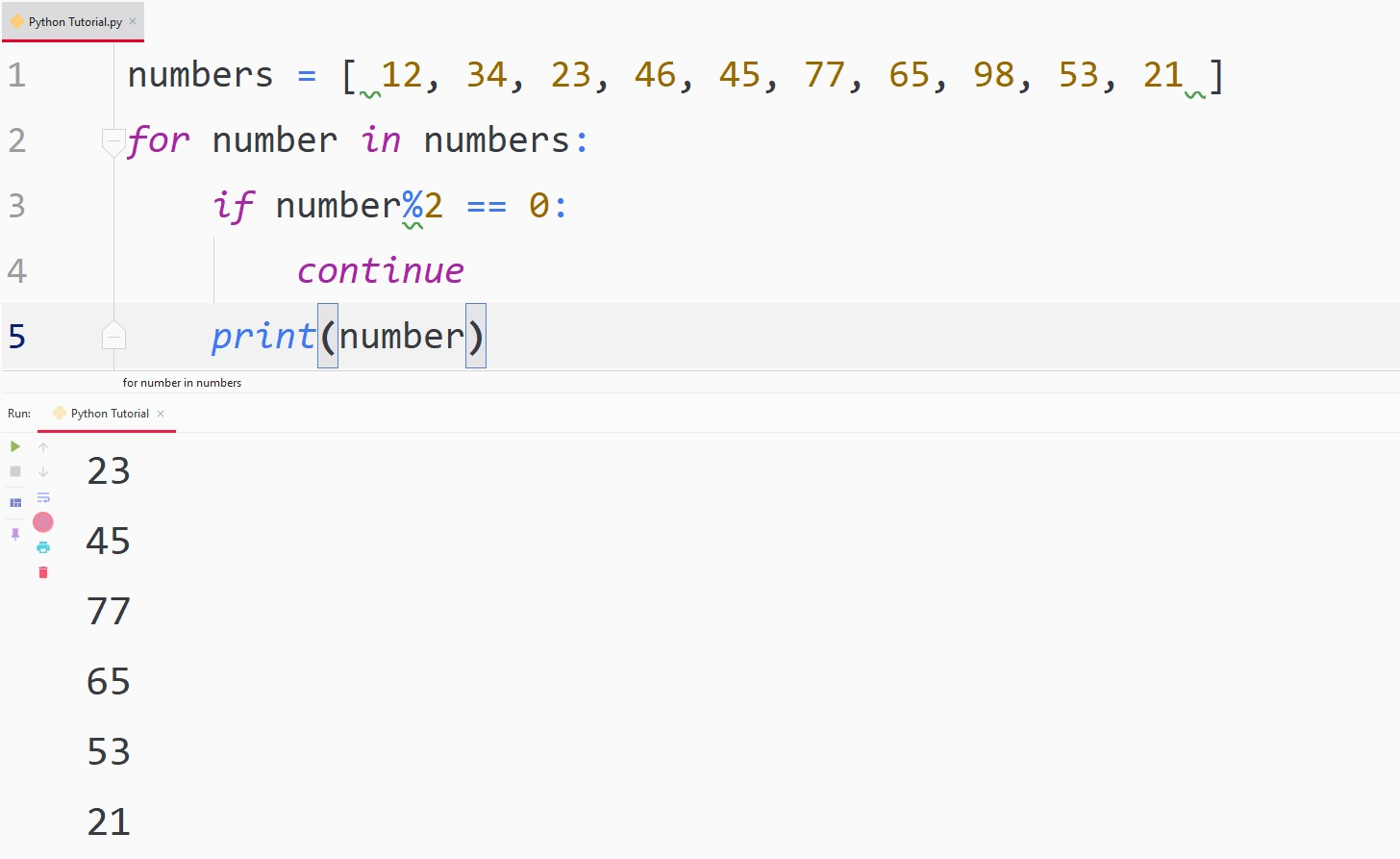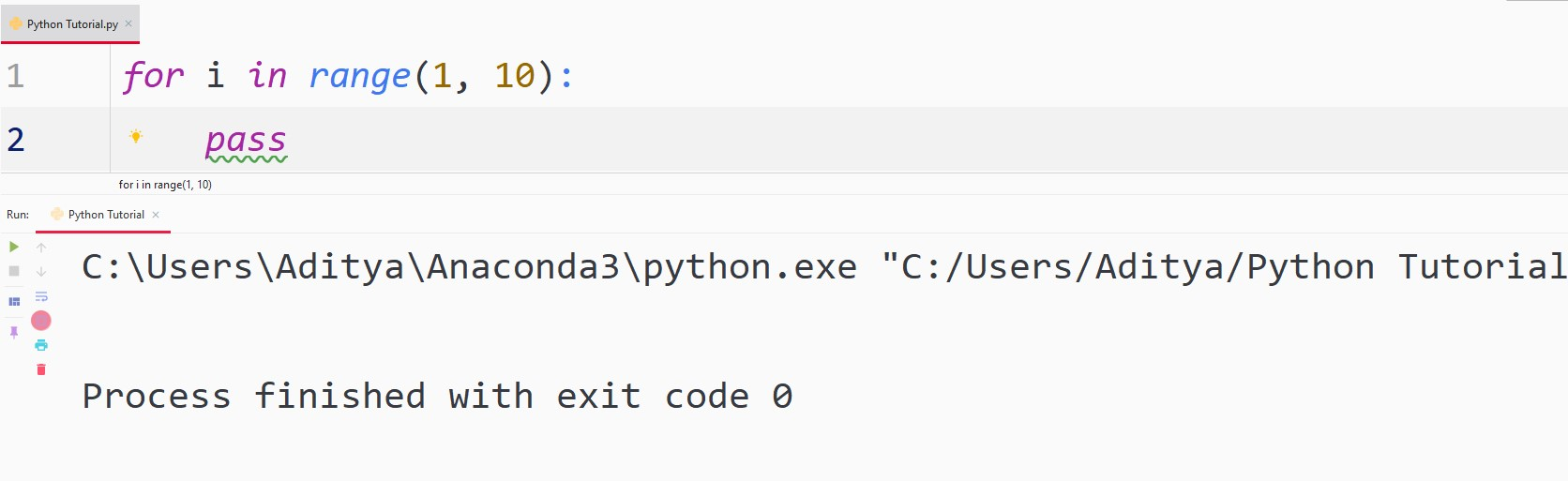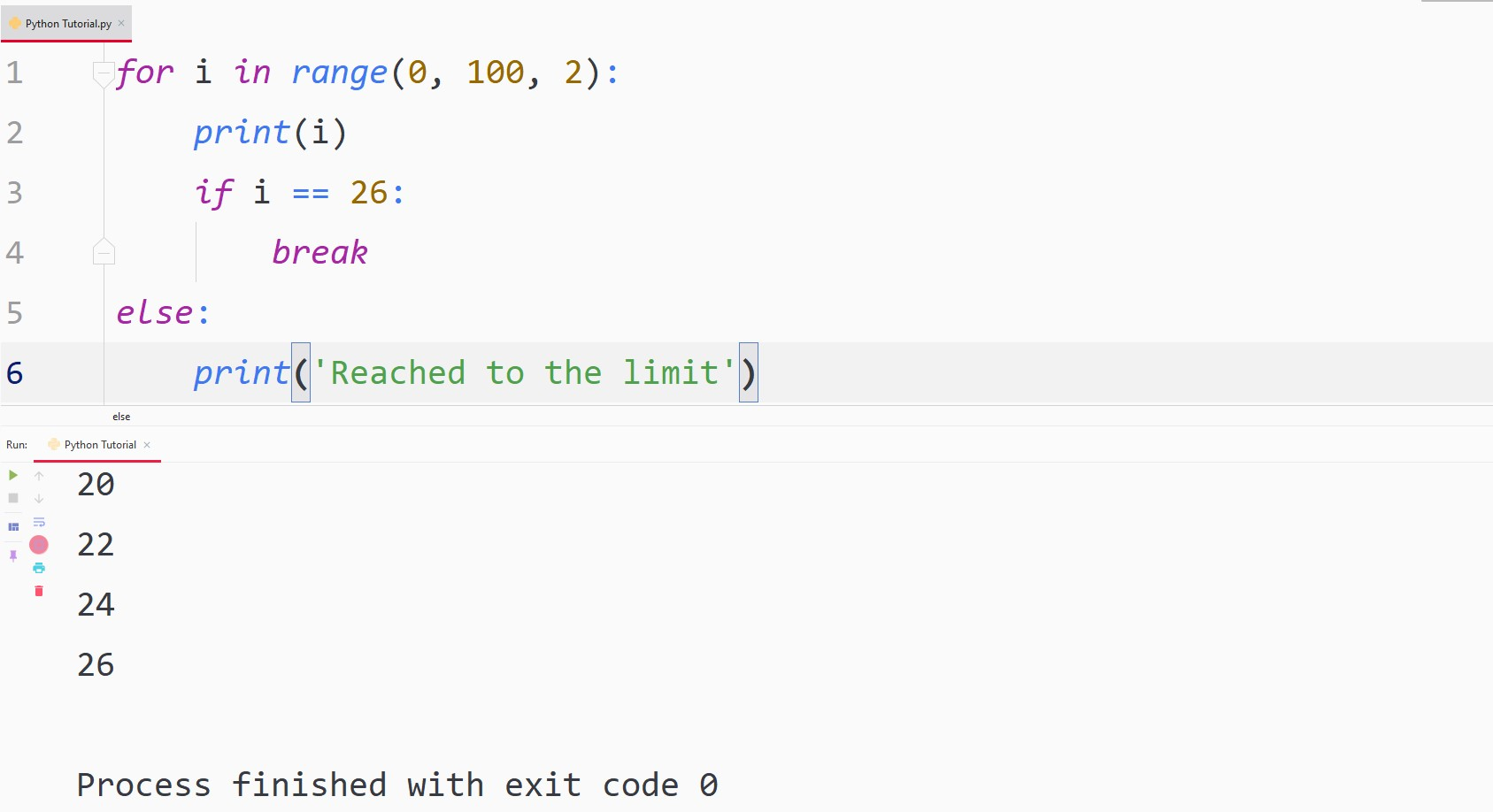# Python For Loop

## Python For Loop

A for loop in Python takes a group or sequence of items and then executes a piece of code for each item in the group. The sequence can be either a tuple, a list, a string, or a tuple.

## ExampleLet’s analyze the execution of the code above step by step –

1. We defined a List of numbers from 1 to 10 and gave the List a name ‘numbers’.
2. Then we defined a for loop and passed our List into it. The line “for number in numbers” basically tells Python to iterate through all of the elements in ‘numbers’. Here, ‘number’ is called an iterator variable and the user can write according to their wish.
3. Now, we write a simple code to print every number in the List. Basically, Python is going through every element in the List and printing it.

Tips
A important thing to keep in mind while writing code is that you could have written “for x in numbers” instead of “for number in numbers” and the code would have worked without a flaw, but it is recommended to make the code more readable, thus we wrote, “for number in numbers”.

Syntax in general form-
for iter_var in sequence:
Code to be executed

## List Comprehension using For Loop

We can use for loop in a single line to do many operations on a List –

## ExampleThis is a little bit complex but it makes the code looks smaller and cleaner, let’s understand the alternative syntax above:

1. We define a List and call it ‘numbers’.

2. Now, in a single line we write the code to square every number in the List. Note that the iterator variable is written before we actually declare it

## ExampleThe code above cubes each term and prints it individually.

## range() Function

The range() function generates a sequence of numbers.

## ExampleThe function range(10) generates a sequence of numbers that starts from 0 and increments 1 by default and ends at 9.

We can, however, change the default values according to our needs, let’s have a look at how that can be done:

## ExampleAbove, the first argument is the starting limit of the range function, and the second argument is the end limit.

This code still increments the sequence by 1, this function gives us the ability to change that too!

## ExampleTo do custom increment we included a third argument, thus we can write the general syntax of the range() function like this:

Syntax in general form-
range(starting_value, end_value, increment_value)

## continue And pass Statements

continue Statement:

The continue statement returns the interpreter to the starting of the for loop and executes the next cycle.

Let us write a code to only print odd numbers from the list of random numbers:

## ExampleAs you can see in the output, we only got odd numbers, because whenever the condition ‘number%2 == 0’ became true, due to continue statement, the interpreter returned to the starting of the for loop and executed the next iteration.

pass Statement:

If you will try to leave a for loop empty, Python will give you an error. But let’s say for some reason, you want to leave it empty, it can be done with the help of a pass statement. Let us have an example to understand it better:

## ExampleAbove, Python didn’t give any error and executed the code block successfully.

## For Loop and Else

We can also include an else statement after the for loop, it will only execute if the for loop ends.

## ExampleYou might ask that the else statement will be executed in each and every code but the execution of the else statement can be prevented using the break statement. Let us have a look:

## ExampleIn the code above, the else block didn’t get executed.